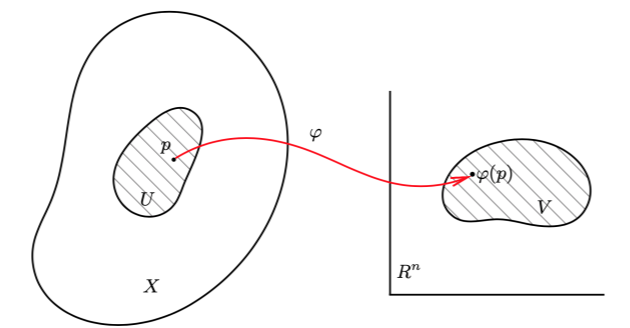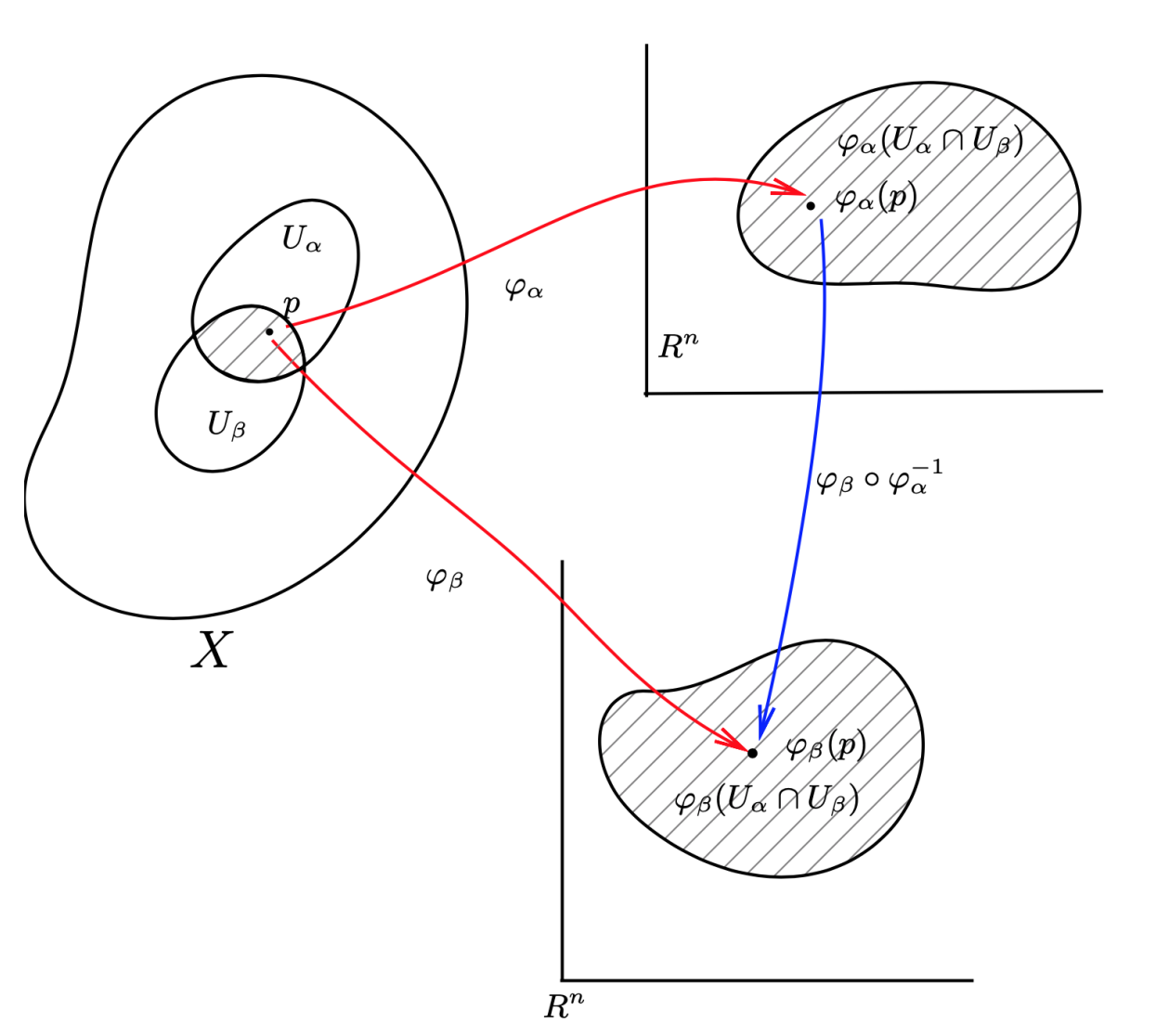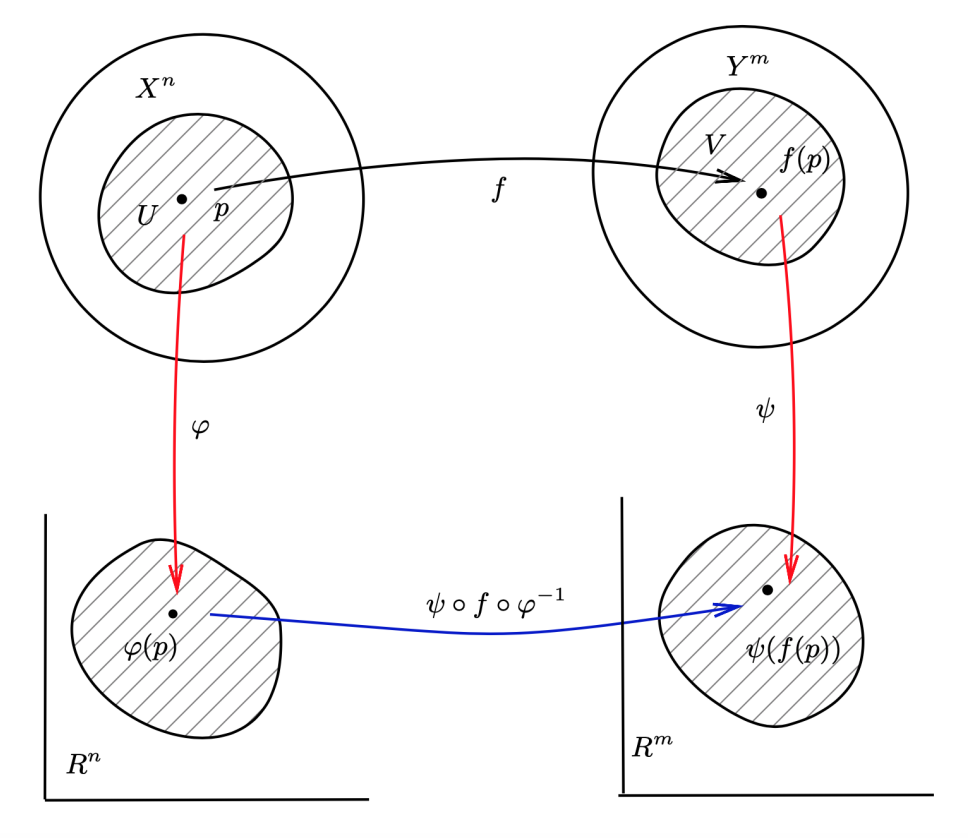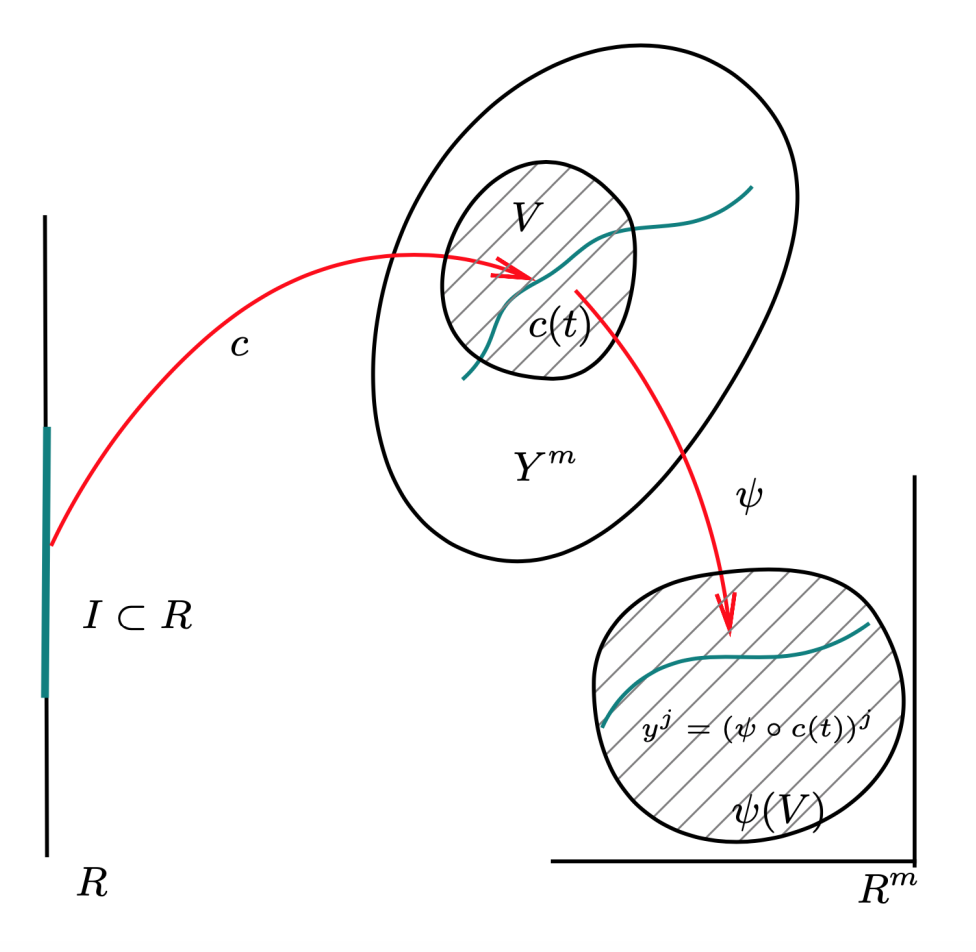## 拓扑流形

$X$ 是一个 Hausdorff 拓扑空间，$\forall p\in X$，都有 $p$ 的一个邻域 $N(p)$ 同胚于 $\mathbb{R}^n$ 中的某个开集。则称 $X$ 为一个 $n$-维的拓扑流形（或简称流形）。$p \in U$，则 $\varphi(p) \in V \subset \mathbb{R}^n$ 。在 $\mathbb{R}^ n$$\varphi(p)$ 可以用一组数

$(x^1 (p),\cdots ,x^ n (p))$

\begin{aligned} &x^i : \mathbb{R}^n \rightarrow \mathbb{R}\\ &u \mapsto x^i (u) = (u)^i ,\ \forall u \in \mathbb{R}^n \end{aligned}

$x_\varphi^i=x^i\circ \varphi:\quad p\mapsto x_\varphi^i(p)=(\varphi(p))^i$

## 微分流形

### 坐标卡集与微分结构

• $\{U_\alpha \}$$X$ 的一个覆盖
• 相容性条件
由同胚 $\varphi_ \alpha$ 给出的映射

$\varphi_\beta \circ \varphi^{-1}_\alpha: \varphi _\alpha (U_ \alpha \cap U_\beta ) \rightarrow \varphi_{\beta} (U_{\alpha} \cap U_{\beta} )$

$\mathbb{R}^n$ 中开集与开集间的 $C^k$ 类（同胚）映射，即：

$x^i \mapsto y^i = y^i (x_1 ,\cdots ,x_n )$

$n$$C^k$ 类函数。这时，我们称这两个坐标卡是 $C^k$-相容的。$X$ 是一个 $n-$ 维流形，$\mathscr{A} = \{(U_{\alpha} ,\varphi_{\alpha} )\}$ 是它的一个坐标卡集，并满足下面条件：

• $\{U_\alpha \}$$X$ 的一个开覆盖；
• $\mathscr{A}$ 中的任意两个坐标卡是 $C^k$-相容的；
• $\mathscr{A}$ 是极大的，即若 $(U,\varphi)$$X$ 的任一个坐标卡且和 $\mathscr{A}$ 中每个坐标卡都是 $C^k$-相容，那么它必然属于 $\mathscr{A}$

### 直积流形

$\varphi_Z(p,q) = (\varphi_X(p),\varphi_Y(q)),\quad \forall\ (p,q)\in U_Z$

## 可微映射

### 可微函数

$F = f \circ \varphi^{-1}$

$\varphi(p)$ 是可微的。

$f\circ \tilde{\varphi}^{-1} = (f\circ \varphi^{-1})\circ (\varphi\circ \tilde{\varphi}^{-1})$

$r$ 阶可微函数

$\varphi \circ \tilde{\varphi} ^{-1}$$C^ k$ 的，所以若想得到坐标卡不依赖的定义，我们要求 $r \leqslant k$

$C^r$ 类函数

### 可微映射$X^n$$Y^m$ 是两个 $C^k$ 微分流形，$f$ 是一个映射：$f : X^n \rightarrow Y^m$。设 $(U,\varphi)$$X_ n$ 的一个坐标卡，而 $(V,\psi)$$Y_n$ 的一个坐标卡，则映射 $\mathcal{F}$

$\mathcal{F} = \psi \circ f \circ \varphi ^{-1}$

$y^I = \mathcal{F}^I(x^1,\cdots,x^n),\quad I=1,\cdots,m$

$m$$C^r$ 个可微函数，其中 $\{x^i,i=1,\cdots,n\}$ 是点 $p$ 的坐标，而 $y^I,I=1,\cdots,m$ 是点 $f(p)$ 的坐标。

• 若取 $Y^m$$\mathbb{R}$，得到了我们前面有关可微函数的定义。
• 若取 $X^n$$\mathbb{R}$ 中的一个区间 $I$，则我们得到了 $Y^m$ 上的 $C^r$ 类参数曲线。

$X$ 是一个 $n$-维微分流形，映射 $c : I \rightarrow X , t \mapsto c(t)$ 称为 $X$ 的一条曲线。在 $X$ 的坐标卡 $(U,\varphi)$ 下，该曲线可表示为：

$x ^ i = (\varphi \ \circ\ c(t))^ i ,\quad i = 1,\cdots ,n$

$x^i = c^i(t) \ or\ x^i = x^i(t)$$X^n$$Y^n$$C^k$ 微分流形。若存在一个映射 $f : X \rightarrow Y$ 满足：

• $f$ 是双射
• $f$$f^{-1}$ 都是 $C^ r$ 可微的

\begin{aligned} &(f_ 1 + f_ 2 )(p) = f_ 1 (p) + f_ 2 (p), (λf)(p) = λf(p)\\ &\qquad \forall f_1 ,f_ 2 \in \mathscr{F}(X),\ \forall p \in X ,\ \forall \lambda \in \mathbb{R}\\ \end{aligned}

$(f_ 1 f_ 2 )(p) = f_ 1 (p)f_ 2 (p)$

$\Phi : X \rightarrow Y$ 是一个光滑映射。则映射 $\Phi$ 诱导出 $\mathscr{F}(Y )$$\mathscr{F}(X)$ 的映射 $\Phi^*$ 。若 $g \in \mathscr{F}(Y )$$\Phi^* g$ 定义为 (映射的复合)：

$(\Phi^* g)(p) = g(\Phi(p)) = g \circ \Phi(p)$

## 微分结构和群结构

$G \times G \rightarrow G, (x,y) \mapsto xy^{-1}$